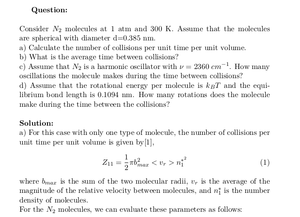Solved Problems in Physics, Physical Chemistry, and Computer Simulation Projects for College and Graduate Students.# Molecular Collision

Regular price
\$0.00
Sale price
\$0.00
Tax included.

Question:

Consider N2  molecules at 1 atm and 300 K. Assume that the molecules are spherical with diameter d=0.385 nm.
a) Calculate the number of collisions per unit time per unit volume.
b) What is the average time between collisions?
c) Assume that N2 is a harmonic oscillator with ν = 2360 cm -1 . How many oscillations the molecule makes during the time between collisions?
d) Assume that the rotational energy per molecule is kBT and the equilibrium bond length is 0.1094 nm. How many rotations does the molecule make during the time between the collisions?

By purchasing this product, you will get the step by step solution of the above problem in pdf format and the corresponding latex file where you can edit the solution. I am also available to help  you with any possible question you may have.